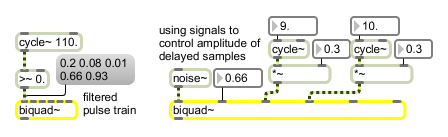Two-pole, two-zero filter

## Description

biquad~ implements a two-pole, two-zero filter using the following equation:

y[n] = a0 * x[n] + a1 * x[n-1] + a2 * x[n-2] - b1 * y[n-1] - b2 * y[n-2]

You can specify the coefficients a0, a1, a2, b1, and b2 as signals or floats (if you make the filter explode by making the b coefficients too high, you can recover (after lowering them) with the clear message, or by turning the audio on and off). .

## Arguments

Name Type Opt Description
filter-parameters (5 floats) list opt Up to five numbers, to set initial values for the coefficients a0, a1, a2, b1, and b2. If a signal is connected to a given inlet, the coefficient supplied as an argument for that inlet is ignored.

## Messages

 int filter-coefficient [int] Converted to float. float filter-coefficient [float] The coefficients in inlets 2 to 6 may be specified by a float instead of a signal. If a signal is also connected to the inlet, the float is ignored. list filter-coefficients (5 floats) [list] The five coefficients can be provided as a list in the left inlet. The first number in the list is coefficient a0, the next is a1, and so on. If a signal is connected to a given inlet, the coefficient supplied in the list for that inlet is ignored. clear Clears the biquad~ object's memory of previous inputs and outputs, resetting xn-1, xn-2, yn-1, and yn-2 to 0. signal In left inlet: Signal to be filtered. The filter mixes the current input sample with the two previous input samples and the two previous output samples according to the formula: yn = a0xn + a1xn-1 + a2xn-2 - b1yn-1 - b2yn-2. In 2nd inlet: Amplitude coefficient a0, for scaling the amount of the current input to be passed directly to the output. In 3rd inlet: Amplitude coefficient a1, for scaling the amount of the previous input sample to be added to the output. In 4th inlet: Amplitude coefficient a2, for scaling the amount of input sample n-2 to be added to the output. In 5th inlet: Amplitude coefficient b1, for scaling the amount of the previous output sample to be added to the current output. In right inlet: Amplitude coefficient b2, for scaling the amount of output sample n-2 to be added to the current output. . stoke filter coefficient values [list] The word stoke, followed by a list that specifies a set of filter coefficient values, will preload the filter so that it starts in a given initial state, allowing the filter to be used for purposes such as a ringing oscillator.

## Attributes

Name Type g/s Description
smooth int
def.:1
Toggles the smoothing of filter coeffient changes. .

## Output

signal: The filtered signal.

## ExamplesFilter coefficients may be supplied as numerical values or as varying signals